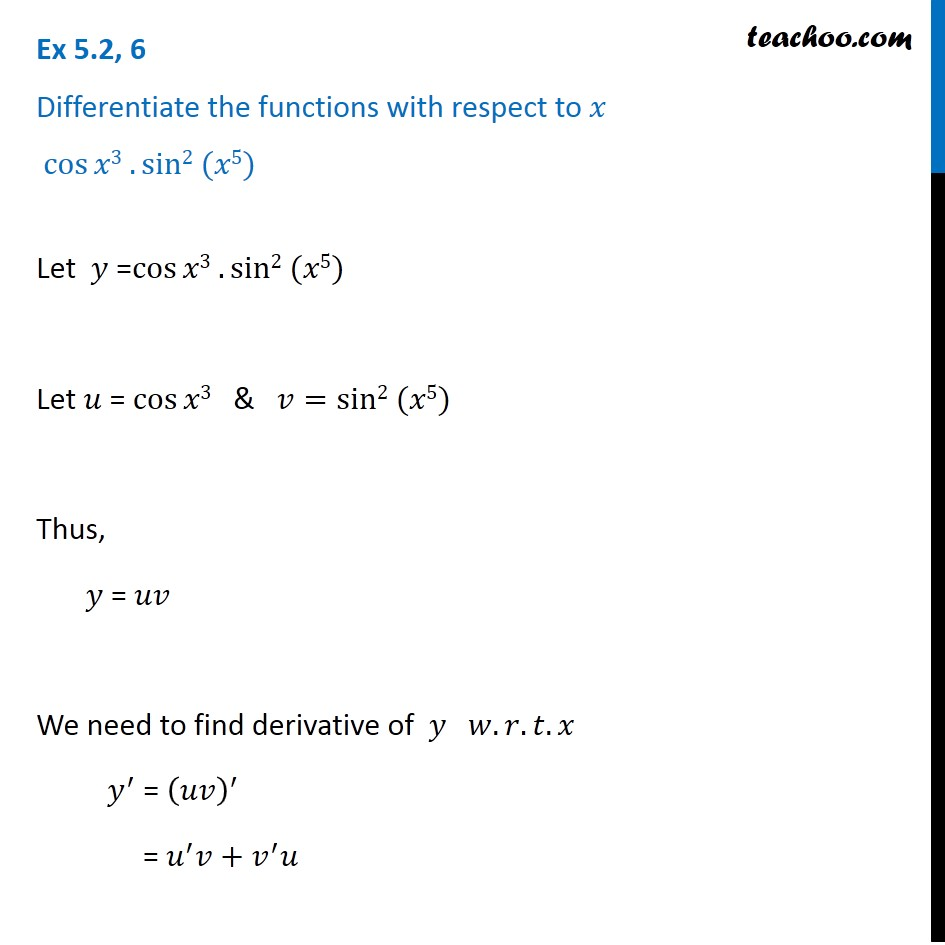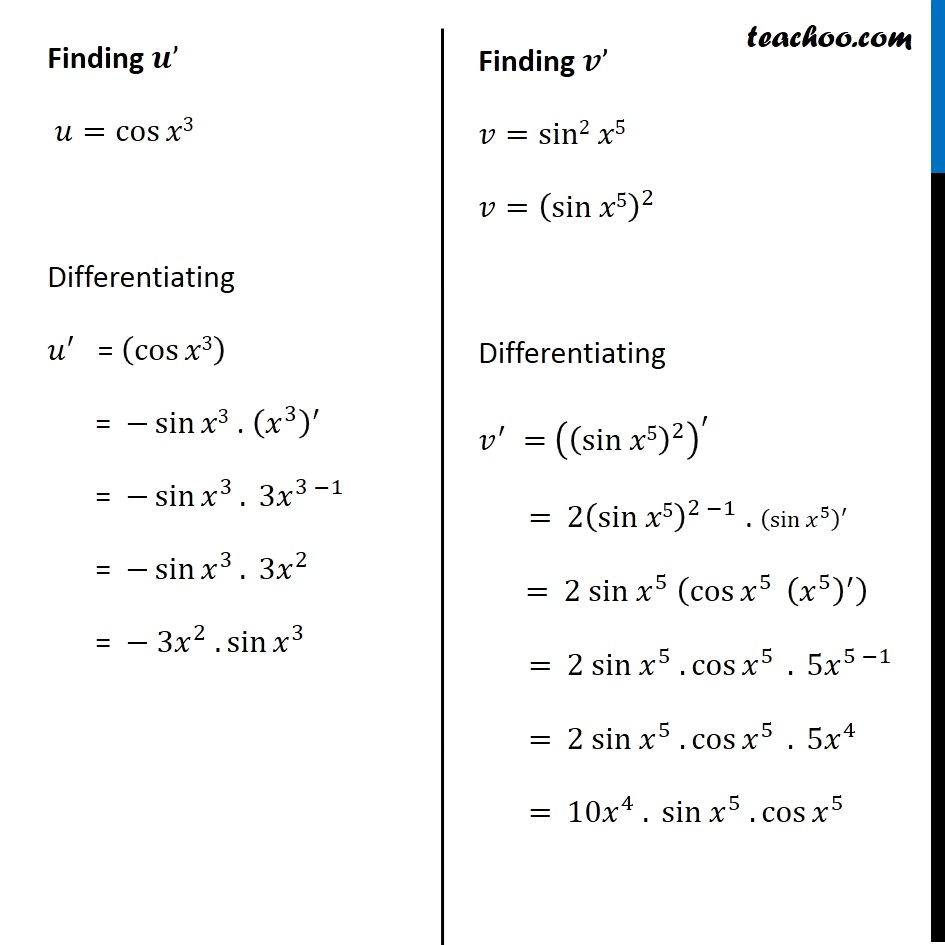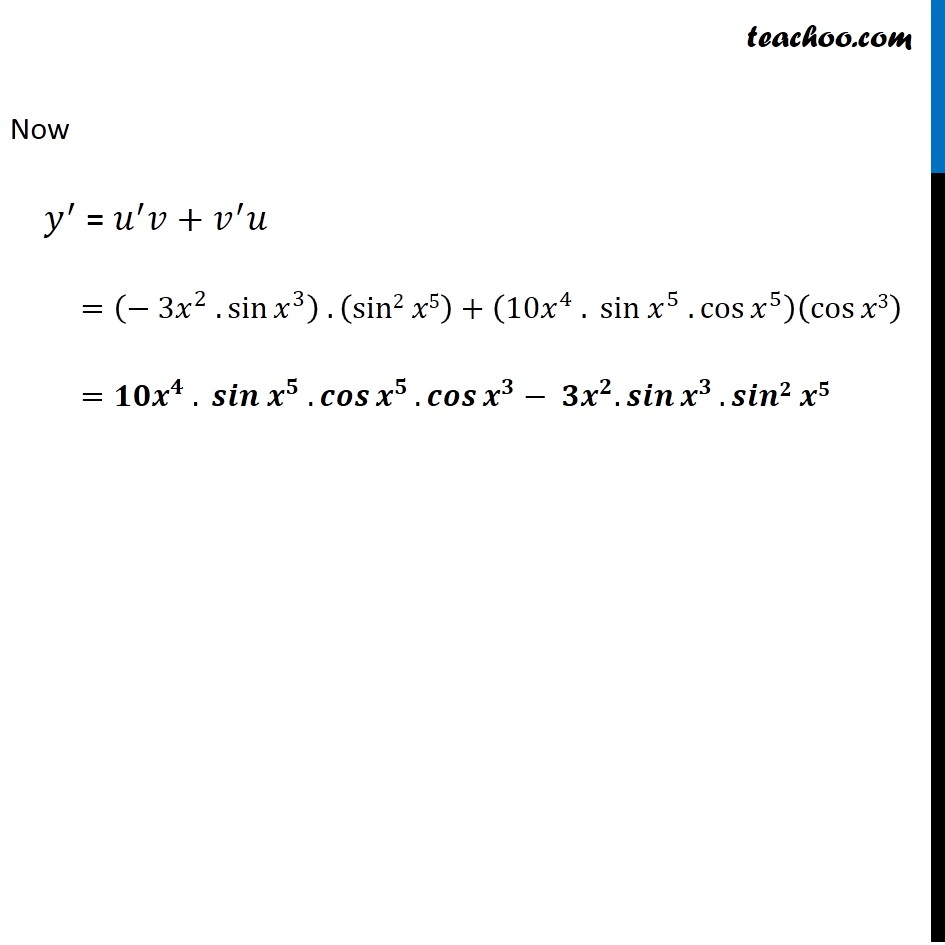Check Full Chapter Explained - Continuity and Differentiability - Continuity and Differentiability Class 121. Chapter 5 Class 12 Continuity and Differentiability
2. Serial order wise
3. Ex 5.2

Transcript

Ex 5.2, 6 Differentiate the functions with respect to 𝑥 cos⁡𝑥3 . sin2 (𝑥5) Let 𝑦 =cos⁡𝑥3 . sin2 (𝑥5) Let 𝑢 = cos⁡𝑥3 & 𝑣=sin2 (𝑥5) Thus, 𝑦 = 𝑢𝑣 We need to find derivative of 𝑦 𝑤.𝑟.𝑡.𝑥 𝑦^′ = (𝑢𝑣)^′ = 𝑢^′ 𝑣+𝑣^′ 𝑢 Finding 𝒖’ 𝑢=cos⁡𝑥3 " " Differentiating 𝑢^′ = (cos⁡𝑥3) = −sin⁡𝑥3 . (𝑥^3 )^′ = −sin⁡〖𝑥^3 〗. 3𝑥^(3 −1) = −sin⁡〖𝑥^3 〗. 3𝑥^2 = − 3𝑥^2 . sin⁡〖𝑥^3 〗 Finding 𝒗’ 𝑣=sin2 𝑥5 𝑣=(sin 𝑥5)^2 Differentiating 𝑣^′ = ((sin 𝑥5)^2 )^′ = 2(sin 𝑥5)^(2 −1) . (sin 𝑥^5 )^′ = 2 sin 𝑥^5 (cos⁡〖𝑥^5 〗 (𝑥^5 )^′ ) = 2 sin 𝑥^5 .cos⁡〖𝑥^5 〗 . 5𝑥^(5 −1) = 2 sin 𝑥^5 .cos⁡〖𝑥^5 〗 . 5𝑥^4 = 10𝑥^4 . sin 𝑥^5 .cos⁡〖𝑥^5 〗 Now 𝑦^′ = 𝑢^′ 𝑣+𝑣^′ 𝑢 =(− 3𝑥^2 . sin⁡〖𝑥^3 〗 ) .(sin2 𝑥5)+(10𝑥^4 . sin 𝑥^5 .cos⁡〖𝑥^5 〗 )(cos⁡𝑥3) =𝟏𝟎𝒙^𝟒 . 𝒔𝒊𝒏 𝒙^𝟓 .𝒄𝒐𝒔⁡〖𝒙^𝟓 〗. 𝒄𝒐𝒔⁡〖𝒙^𝟑 〗− 𝟑𝒙^𝟐. 𝒔𝒊𝒏⁡〖𝒙^𝟑 〗.𝒔𝒊𝒏𝟐 𝒙𝟓

Ex 5.2